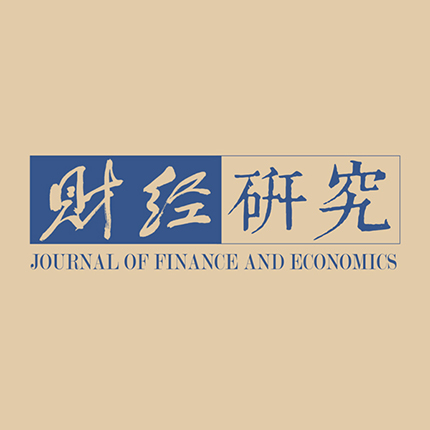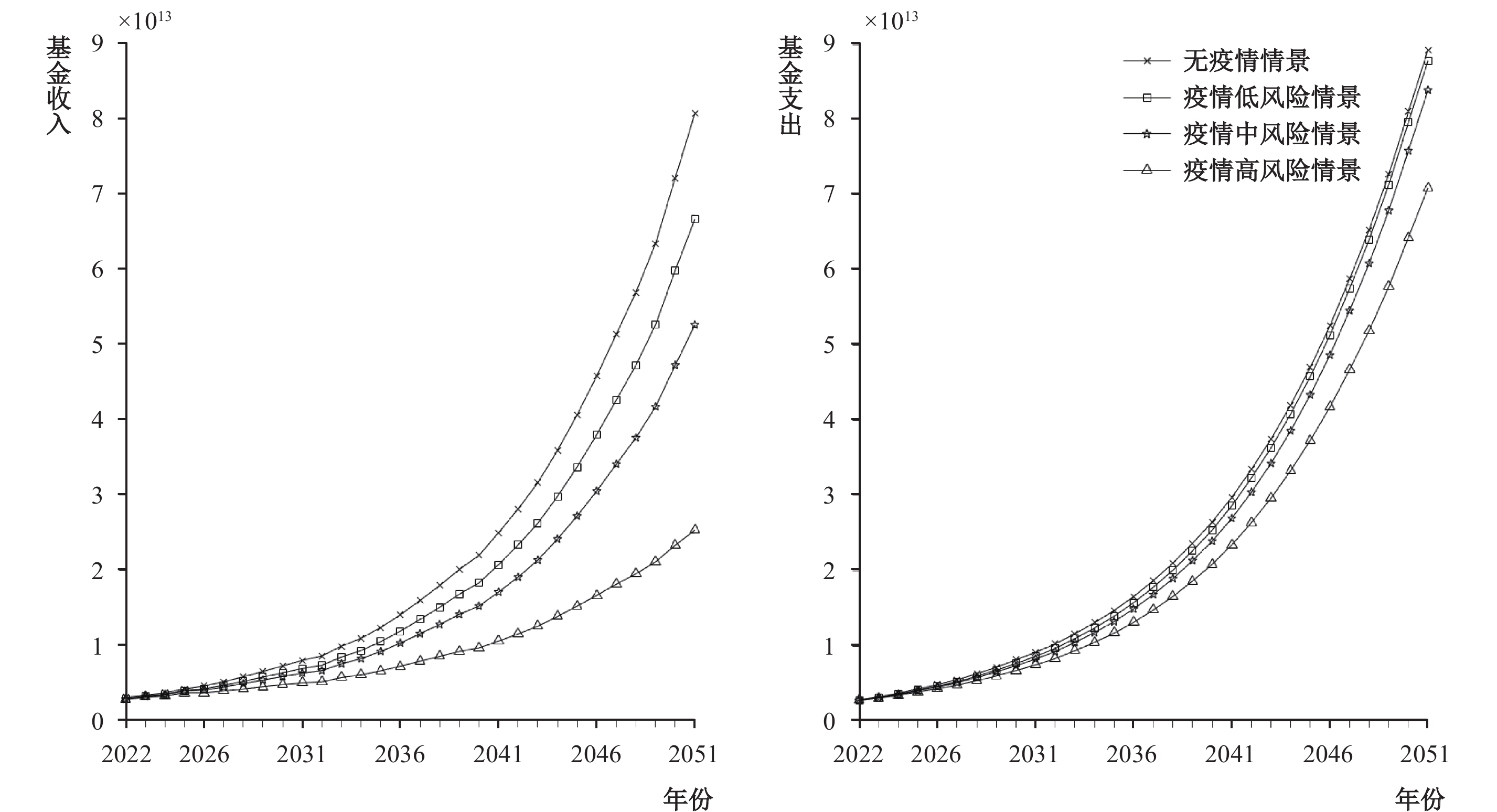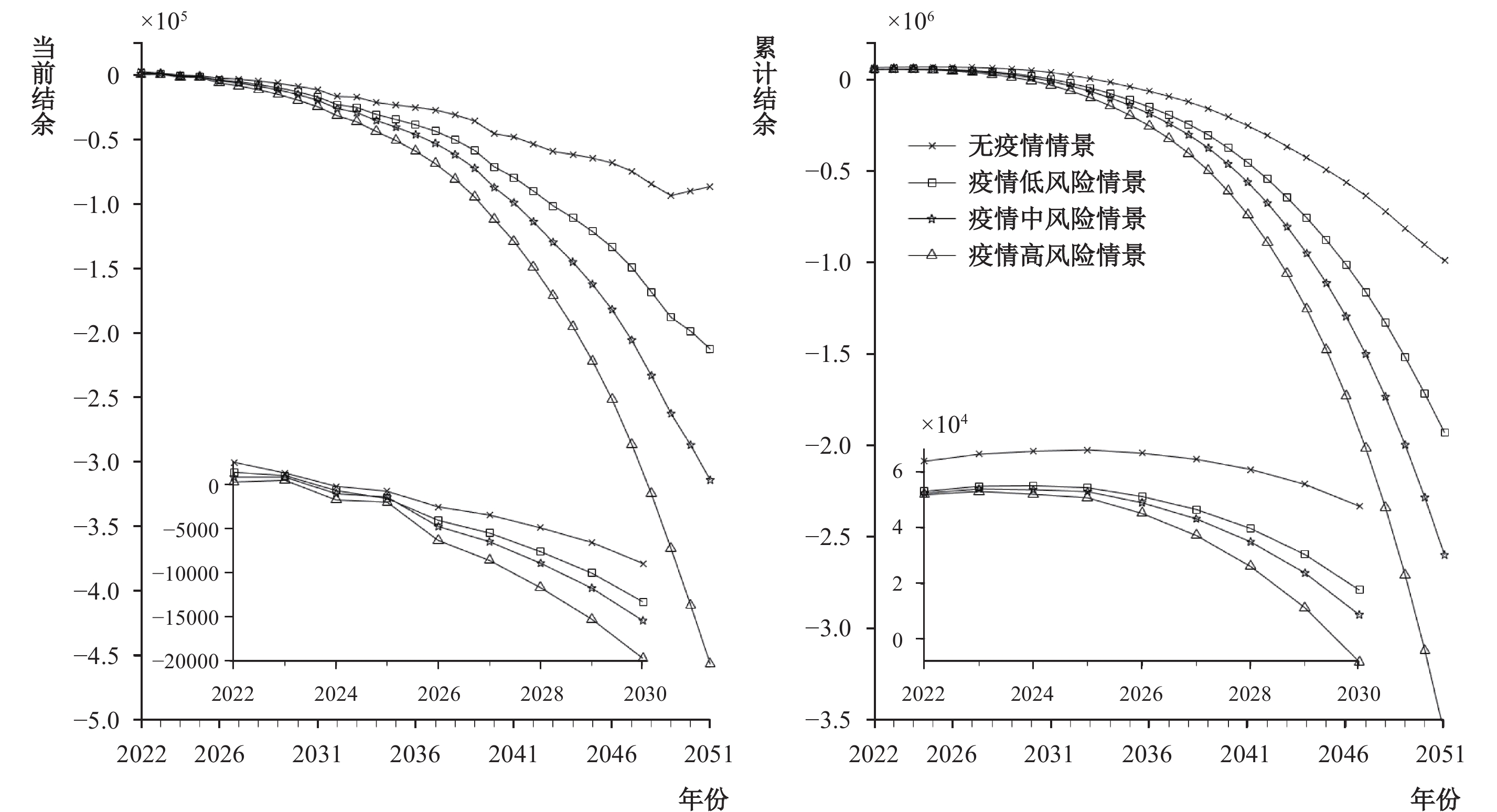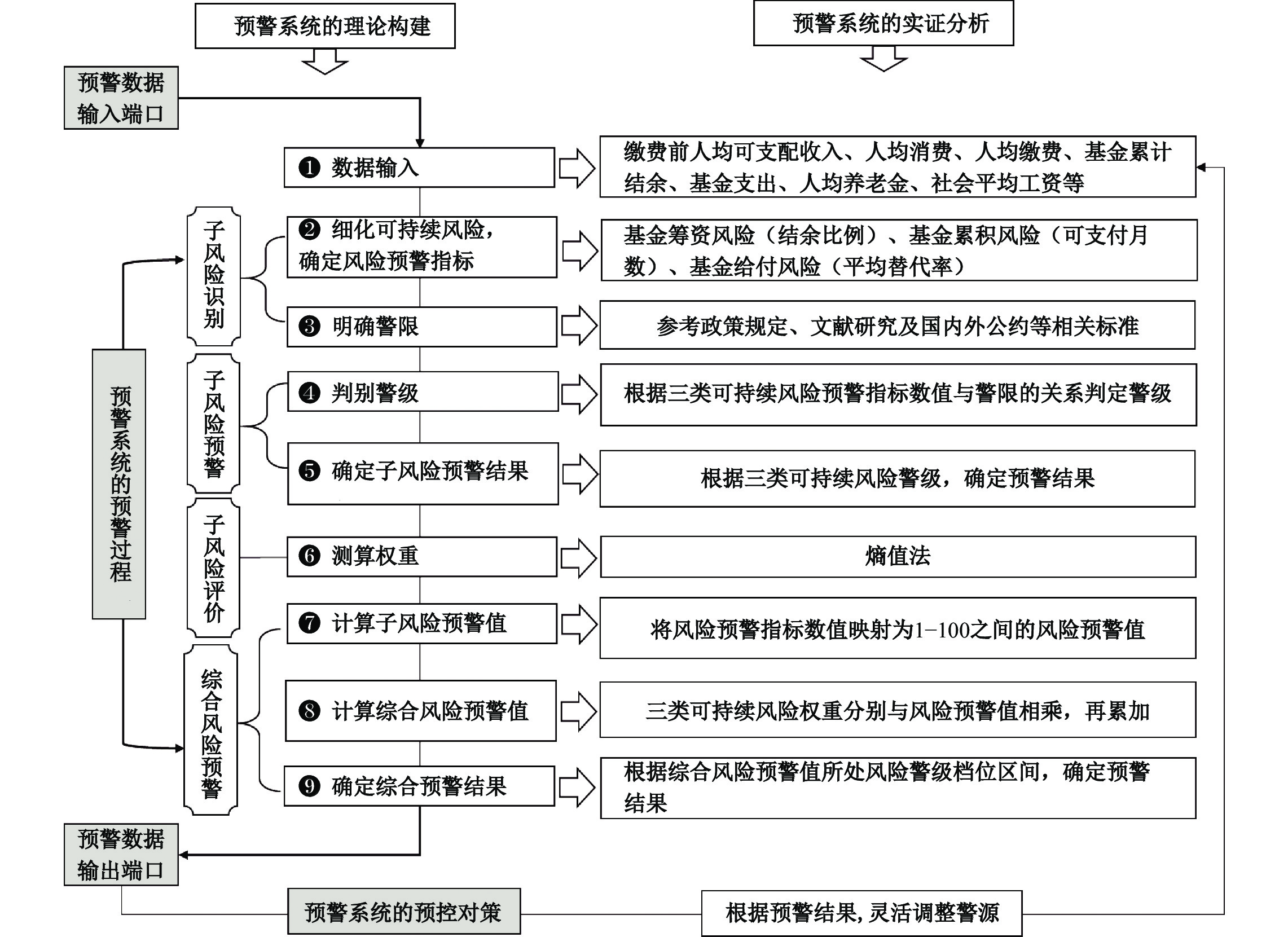﻿ 企业职工基本养老保险基金收支预测与可持续风险监测预警《财经研究》
2023第49卷第3期

Income-Expenditure Forecast and Sustainable Risk Monitoring and Warning on the Fund of the Basic Pension Insurance for Enterprise Employees: Scenario Analysis Based on the Impact of the COVID-19 Epidemic
Huang Xiulian, Chu Fuling
School of Insurance, Central University of Finance and Economics, Beijing 100081, China
Summary: The sustainability of fund for the basic pension insurance for enterprise employee is weakening in China with the current income and expenditure gap on the fund expanding year by year.Under different epidemic scenarios including epidemic-free scenario, low-risk scenario, medium-risk scenario and high-risk scenario, we use the econometric model and actuarial model to measure the income and expenditure balance of the fund of the basic pension insurance for enterprise employees from 2022 to 2051, and build a sustainable risk monitoring and warning system. The results show that: First, for each increase in the risk level of the COVID-19 epidemic impact, the cumulative present value of fund income during the measurement period decreases by an average of 23.45%, the cumulative present value of fund expenditure decreases by an average of 7%, and the sum of the cumulative present value of fund balance decreases by an average of 59.76%. Second, under the impact of the COVID-19 epidemic, if effective measures are not taken, the cumulative balance of the basic pension insurance fund for enterprise employees will be exhausted range from 2030 to 2032, and the scale of the deficit will increase year by year. Third, this paper builds a sustainable risk monitoring and warning system by linking fund-raising risk, fund-accumulation risk and fund-payment risk. The marginal contribution is as follows: First, this paper reasonably assesses the impact of the COVID-19 epidemic on fund income and expenditure by the DID method and scenario analysis. Second, this paper establishes a monitoring and warning system for the sustainable risk of pension fund in a trinity of “raising, accumulation and payment”. It not only clarifies the theoretical construction of warning system, but also performs empirical analysis. Third, this paper proposes a risk prevention and control system of “forecast, warning and pre-control”. Based on the forecast results of fund income and expenditure, it analyzes the sustainable risk monitoring and warning system, and develops the pre-control countermeasure through adjusting warning sources. This paper not only improves the pension fund risk prevention and control system, but also provides reference for effectively dealing with preventing and defusing major risks.
Key words: fund income-expenditure forecast    sustainable risk    risk monitoring and warning    the COVID-19 epidemic

（一）基金收入模型

 ${{AI}}_{{t}}=\left[\left(\sum _{{i=}{1}}^{{3}}\sum _{{x=}{{a}}_{{i}}}^{{{r}}_{{i}}-{1}}{{N}}_{{t,x}}^{{i}}\right){\stackrel{\mathrm-}{{w}}}_{{t}}{{d}}_{{1}}\left({{R}}_{{i,t}}^{{1}}+{{R}}_{{i,t}}^{{2}}\right)+\left(\sum _{{j=}{1}}^{{2}}\sum _{{x=}{{a}}_{{j}}}^{{{r}}_{{j}}-{1}}{{N}}_{{t,x}}^{{j}}\right){\stackrel{\mathrm-}{{w}}}_{{t}}{{d}}_{{2}}\left({{R}}_{{j,t}}^{{1}}+{{R}}_{{j,t}}^{{2}}\right)\right]\cdot{\alpha}_{{t}}\cdot{\partial }_{{t}}$ (1)

（二）基金支出模型

 ${{AE}}_{{t}}={{AEL}}_{{t}}+{{AEZ}}_{{t}}+{{AEX}}_{{t}}+{{AEI}}_{{t}}^{D}$ (2)

1. “老人”养老金支出

 ${{AEL}}_{{t}}=\sum _{{x=r+t-z}}^{{ \omega }}{{N}}^L_{{t,x}}{{P}}_{{t,x}}=\sum _{{x=r+t-z}}^{{ \omega }}{{N}}^L_{{t,x}}{{(1}{+b}{)}}^{{x-r}}{{R}}_{{t}}{{S}}_{{t-}{1}{,r-}{1}}=\sum _{{x=r+t-z}}^{{ \omega }}{{N}}^L_{{t,x}}{{{(1}{+b}{)}}^{{x-r}}{R}}_{{t}}{{(1}{+s}{)}}^{{r-}{1}{-e}}{{S}}_{{t,e}}{/}{(1}{+g}{)}$ (3)

2. “中人”养老金支出

 \begin{aligned} {{AEZ}}_{{t}}= &\sum _{{x=r+t-z}}^{{ \omega }}{{N}}^Z_{{t,x}}{{B}}_{{t,x}}^{{z}}+\sum _{{x=r+t-z}}^{{ \omega }}{{N}}^Z_{{t,x}}{{T}}_{{t,x}}^{{z}}+\sum _{{x=r+t-z}}^{{ \omega }}{{N}}^Z_{{t,x}}{{I}}_{{t,x}}^{{z}} \\ =&\sum _{{x=r+t-z}}^{{ \omega }}{{N}}^Z_{{t,x}}\left\{\frac{{\stackrel{\mathrm-}{{S}}}_{{t-}{(}{x-r}{)}-{1}}}{{2}}\left[{1}+\frac{{\partial}_{{t}}{d}}{{t-}{(}{x-r}{)}{-z}}\sum _{{k=}{1}}^{{t-}{(}{x-r}{)}{-z}}\frac{{{S}}_{{t-}{(}{x-r}{)}{-k-}{1}{,r-k-}{1}}}{{\stackrel{\mathrm-}{{S}}}_{{t-}{(}{x-r}{)}{-k-}{1}}}\right]{[}{t-}{(}{x-r}{)}{-z}{]}{\%}\frac{\prod _{{h=t-}{(}{x-r}{)}}^{{t}}{1}+{\rho}_{{h}}}{{1}+{\rho}_{{t}}}\right\}\\& +\sum _{{x=r+t-z}}^{{ \omega }}{{N}}^Z_{{t,x}}\left\{\frac{{\stackrel{\mathrm-}{{S}}}_{{t-}{(}{x-r}{)}-{1}}{\partial}_{{t}}{d}}{{t-}{(}{x-r}{)}{-z}}\left[\sum _{{k=}{1}}^{{t-}{(}{x-r}{)}{-z}}\frac{{{S}}_{{t-}{(}{x-r}{)}{-k-}{1}{,r-k-}{1}}}{{\stackrel{\mathrm-}{{S}}}_{{t-}{(}{x-r}{)}{-k-}{1}}}\right]{ \varepsilon }{\left\{{}{r-e-}{[}{t-}{(}{x-r}{)}{-z}{]}\right\}{}}{\%}\frac{\prod _{{h=t-}{(}{x-r}{)}}^{{t}}{1}+{\rho}_{{h}}}{{1}+{\rho}_{{t}}}\right\} \\& +\sum _{{x=r+t-z}}^{{ \omega }}{{N}}^Z_{{t,x}}\left\{\frac{{12}}{{m}}\frac{{\partial}_{{t}}{d}{{R}}^{{1}}{{S}}_{{z-}{1}{,x-}{[}{t-}{(}{z-}{1)]}}}{{1}+{{g}}_{{z-}{2}}}\sum _{{n=0}}^{{t-}{(}{x-r}{)}{-z-}{1}}\left[\prod _{{k=0}}^{{n}}{(1}+{{g}}_{{z-}{2}{+k}}{)}\prod _{{h=n}}^{{t-}{(}{x-r}{)-}{z-}{1}}{(1}+{{v}}_{{z+h}}{)}\right]\right\} \end{aligned} (4)

3. “新人”养老金支出

 \begin{aligned} {{AEX}}_{{t}}=&\sum _{{x=r+t-z}}^{{ \omega }}{{N}}^X_{{t,x}}{{B}}_{{t,x}}^{{x}}+\sum _{{x=r+t-z}}^{{ \omega }}{{N}}^X_{{t,x}}{{I}}_{{t,x}}^{{x}}\\ =&\sum _{{x=r+t-z}}^{{ \omega }}{{N}}^X_{{t,x}}\left\{\frac{{\stackrel{\mathrm-}{{S}}}_{{t-}{(}{x-r}{)}-{1}}}{{2}}\left[{1}+\frac{{\partial}_{{t}}{d}}{{r-e}}\sum _{{k=}{1}}^{{r-}{e}}\frac{{{S}}_{{t-}{(}{x-r}{)}{-k-}{1}{,r-k-}{1}}}{{\stackrel{\mathrm-}{{S}}}_{{t-}{(}{x-r}{)}{-k-}{1}}}\right]{(}{r-e}{)\%}\frac{\prod _{{h=t-}{(}{x-r}{)}}^{{t}}{1}+{\rho}_{{h}}}{{1}+{\rho}_{{t}}}\right\} \\ &+\sum _{{x=r+t-z}}^{{ \omega }}{{N}}^X_{{t,x}}\left\{\frac{{12}}{{m}}\frac{{\partial}_{{t}}{d}{{R}}^{{1}}{{S}}_{{t-}{(}{r-e}{)}-{1}{,e-}{1}}}{{1}+{{g}}_{{t-}{(}{r-e}{)}-{2}}}\sum _{{n=0}}^{{r-e-}{1}}\left[\prod _{{k=0}}^{{n}}{(1}+{{g}}_{{t-}{(}{r-e}{)}-{2}{+k}}{)}\prod _{{h=n}}^{{r-e-}{1}}{(1}+{{v}}_{{t-}{(}{r-e}{)}{+h}}{)}\right]\right\} \end{aligned} (5)

4. 个人账户余额返还性支出

 ${{AEI}}_{{t}}^{{D}}={\partial}_{{t}}{d}{{R}}^{{1}}\sum _{{x=e}}^{{r-}{1}}{{S}}_{{t-}{1}{,x-}{1}}\sum _{{k=}{1}}^{{x-e+}{1}}{(}\frac{{1}+{{g}}_{{t-}{1}}}{{1}+{{v}}_{{t}}}\prod _{{n=1}}^{{k}}\frac{{1+}{{v}}_{{t-n+}{1}}}{{1+}{{g}}_{{t-n}}}{)}{{D}}_{{t,x}}+\sum _{{x=r}}^{{r}{-[}{m}{/12]-1}}{(}{m}{/}{12-x+r-}{1)}{{I}}_{{t,x}}{{D}}_{{t,x}}$ (6)

（三）当期结余模型

 ${{CS}}_{{t}}={{AI}}_{{t}}-{{AE}}_{{t}}$ (7)

（四）累计结余模型

 ${{AS}}_{{t}}=\left\{\begin{array}{c}{{AS}}_{{t-}{1}}\left({1}+{{\tau }}_{{t-}{1}}\right)+{{CS}}_{{t}}{,}\;{{AS}}_{{t-}{1}}{ > }{0}\\ {{AS}}_{{t-}{1}}+{{CS}}_{{t}}{,}\;{{AS}}_{{t-}{1}}\leqslant {0}\end{array}\right.$ (8)

（一）人口预测

1. 城镇人口结构

2. 灵活就业人员和企业职工的参保人口结构

（二）经济参数

《2021全国毕业生起薪点薪酬报告》中专科毕业生月起薪点为4560元，将此作为2021年全国企业职工新入职人员月平均工资，而正规就业者收入是灵活就业者的1.56倍（薛进军和高文书，2012；张晓昕，2021），那么灵活就业人员新入职月平均工资为2923元。基金保值增值率参考2019年一年期定期存款基准利率与各银行实际存款利率，本文设为2%。工龄工资增长率设为1.58%，高龄养老金增长率设为1.03%（杨再贵和陈肖华，2021），折现率取一年期Shibor同业拆借利率3%。

（三）政策参数

（一）疫情冲击的情景设置

1. 经济参数

（1）经济增长率

 ${{Y}}_{{it}}=\beta _{{0}}+\beta _{{1}}{{treat}}_{{i}}\times {{post}}_{{t}}+{{Z}}_{{it}}^{{'}}\beta _{{2}}+{{u}}_{{i}}+{{v}}_{{t}}+{\xi}_{{it}}{,} \;i = 1, \cdots ,\;N;\;t = 1, \cdots ,\;T$ (9)

 （1） （2） （3） （4） Y Y Y Y treat×post −1.510*** −1.096*** −3.884*** −3.758*** （0.383） （0.358） （0.742） （0.704） Secondind 0.041** 0.087*** （0.018） （0.029） Socialconsum −0.273 −0.245 （0.229） （0.284） Budgetexp 0.171 0.648 （0.379） （0.554） Deposit 2.809*** 3.127** （0.836） （1.236） Loan −0.532 −1.811 （0.609） （1.136） Getechno −0.144 −0.433** （0.131） （0.215） Indprofit 0.546*** 0.468*** （0.124） （0.173） Pop −0.330 0.818 （0.557） （0.689） 常数项 7.047*** −31.130*** 6.678*** −36.960** （0.065） （11.630） （0.089） （16.250） 城市效应 控制 控制 控制 控制 时间效应 控制 控制 控制 控制 样本量 1344 1344 833 833 注：括号内为稳健标准误；*、**和***分别表示10%、5%和1%的显著性水平下显著；列（1）、列（3）为不加控制变量的回归结果，列（2）、列（4）为加上控制变量的回归结果，并且双重差分模型通过了平行趋势检验的建模条件。

（2）就业率

（3）基金保值增值率

2. 人口参数

3. 政策参数

（1）基础养老金增长率

（2）个人账户记账利率

（3）灵活就业人员缓缴比例

《人力资源社会保障部办公厅、国家税务总局办公厅关于特困行业阶段性实施缓缴企业社会保险费政策的通知》（人社厅发〔2022〕16号）指出，以个人身份参加企业职工基本养老保险的个体工商户和各类灵活就业人员可自愿暂缓缴费至2023年底前补缴。由于缺乏缓缴数据，考虑到我国统筹疫情防控和经济社会发展，假设在低、中、高风险情景下2022年灵活就业人员缓缴比例分别为15%、25%和35%，2023年、2024年连续下降5%，2025年恢复正常缴费。

（二）疫情冲击下的基金收支预测图 1 2022—2051年企业职工基本养老保险基金收入和基金支出

（三）疫情冲击下的基金结余预测图 2 2022—2051年企业职工基本养老保险基金当期结余和累计结余

（一）可持续风险预警系统的理论构建图 3 企业职工基本养老保险基金可持续风险监测预警系统

（二）可持续风险预警系统的实证分析

1. 预警数据输入端口

2. 预警系统的预警过程

（1）子风险识别：三类可持续风险预警指标及警限

 可持续风险类别 风险预警区间 基金筹资风险 筹资状态 筹资能力不足 筹资能力较低 筹资能力适中 筹资能力充足 警限 （−∞，0） [0，10%） [10%，25%] （25%，+∞） 警级 重警 中警 轻警 无警 基金积累风险 结余状态 结余过少 结余较低 结余适中 结余充足 警限 （−∞，2） [2，9] （9，15] （15，+∞） 警级 重警 中警 轻警 无警 基金给付风险 给付状态 待遇水平过低 待遇水平偏低 待遇水平适中 待遇水平较高 警限 （−∞，39%） [39%，55%） [55%，70%] （70%，+∞） 警级 重警 中警 轻警 无警

（2）子风险预警：三类可持续风险的预警结果图 4 三类可持续风险预警图

（3）子风险评价：三类可持续风险预警指标权重测算

（4）综合风险预警：综合可持续风险预警

3. 预警结果输出端口

4. 预警系统的预控对策：警源调整

① 文件来源：《国务院关于印发国家人口发展规划（2016—2030年）的通知》（国发〔2016〕87号）。

② 限于篇幅，下文的图表分析省略，读者若是感兴趣可向作者索取。

③ 限于篇幅，基本原理省略，读者若是感兴趣可向作者索取。

④ 数据来源：2018—2020年《中国人力资源和社会保障年鉴》，因缺乏私营企业在岗职工人数，在岗率=非私营企业在岗职工人数/非私营企业就业人数，取2017—2019年平均值92.68%。

⑤ 由《中国统计年鉴》计算1997—2019年缴费基数和企业职工人均养老金平均增速分别为12.16%和9.09%，则9.09%/12.16%=75%。

⑥ 人社部办公厅公布2016—2019年职工基本养老保险个人账户记账利率依次为8.31%、7.12%、8.29%和7.61%，均值为7.83%。

⑦ 基于数据可得性，不包括西藏自治区、中国香港特别行政区、中国澳门特别行政区和中国台湾省，也不包括其他省份中部分数据缺失的城市。

⑧ 在疫情低风险情景下，经济增长率比无疫情情景下降1.37%（8.87%−7.5%=1.37%），故就业率下降0.14%（1.37%×0.103241=0.14%），其他情景类似。

⑨ 缴费前城镇居民人均可支配收入为人均可支配收入与企业职工基本养老保险人均缴费之和。

  褚福灵, 司絮. 突发疫情与城镇职工基本医疗保险基金支付风险预警——基于COVID-19干预下的情景分析[J]. 经济社会体制比较, 2022(2): 85–98. DOI:10.3969/j.issn.1003-3947.2022.02.009  何文炯, 张奕, 徐林荣, 等. 企业职工基本养老保险预警系统初探[J]. 浙江社会科学, 2002(4): 179–181. DOI:10.3969/j.issn.1004-2253.2002.04.036  黄彬云. 城镇非正规服务就业规模的估计[J]. 统计与决策, 2014(23): 37–40.  刘学良. 中国养老保险的收支缺口和可持续性研究[J]. 中国工业经济, 2014(9): 25–37.  卢成会, 吴丽丽. 社会养老保险基金筹资风险的规避研究[J]. 江汉论坛, 2016(3): 17–22. DOI:10.3969/j.issn.1003-854X.2016.03.003  吕有吉, 景鹏, 郑伟. 人口老龄化、养老保险基金缺口弥补与经济增长[J]. 金融研究, 2021(1): 51–70.  任海军, 曹盘龙, 张爽. 基于熵值法的生态社会评价指标体系研究——以我国西部地区为例[J]. 华东经济管理, 2014(5): 71–76.  石晨曦. 企业职工统筹账户自平衡性与财政负担[J]. 求实, 2018(3): 45–57. DOI:10.3969/j.issn.1007-8487.2018.03.004  万磊, 石玉建, 魏栋鸿, 等. 灵活就业人员参保缴费政策评估[J]. 中国社会保障, 2014(6): 36–37. DOI:10.3969/j.issn.1008-4304.2014.06.011  王翠琴, 薛惠元. 新型农村社会养老保险风险预警指标体系的构建[J]. 统计与决策, 2011(16): 28–31.  王翠琴, 岳晓, 薛惠元. 城镇职工基本养老保险基金可持续性仿真测算[J]. 统计与决策, 2021(12): 131–135.  王金营, 戈艳霞. 全面二孩政策实施下的中国人口发展态势[J]. 人口研究, 2016(6): 3–21.  汪泓. 社会保险基金的良性运营: 系统动力学模型、方法、应用[M]. 北京: 北京大学出版社, 2008.  吴成颂. 我国金融风险预警指标体系研究[J]. 技术经济与管理研究, 2011(1): 19–24.  席恒. 全球新冠肺炎疫情、超级老龄化、新型就业三重挑战下的中国社会保障[J]. 社会保障评论, 2022(1): 35–46.  杨钒. 延迟退休对养老金可持续性影响研究[J]. 宏观经济研究, 2020(5): 91–101.  杨再贵, 陈肖华. 降费综合方案下企业职工基本养老保险财政风险预警[J]. 保险研究, 2021(1): 91–106. DOI:10.13497/j.cnki.is.2021.01.006  曾益, 魏晨雪, 李晓琳, 等. 征收体制改革、延迟退休年龄与养老保险基金可持续性——基于“减税降费”背景的实证研究[J]. 公共管理学报, 2019(4): 108–118.  曾益, 杨悦. 从中央调剂走向统收统支——全国统筹能降低养老保险财政负担吗?[J]. 财经研究, 2021(12): 34–48.  张晓昕. 教育、非正规就业与劳动者工资收入——基于CFPS数据的实证分析[J]. 云南财经大学学报, 2021(9): 31–45.  朱恒鹏, 岳阳, 林振翮. 统筹层次提高如何影响社保基金收支——委托-代理视角下的经验证据[J]. 经济研究, 2020(11): 101–120.  Enoff L D, McKinnon R. Social security contribution collection and compliance: Improving governance to extend social protection[J]. International Social Security Review, 2011, 64(4): 99–119. DOI:10.1111/j.1468-246X.2011.01413.x  Hamaaki J, Iwamoto Y. A reappraisal of the incidence of employer contributions to social security in Japan[J]. The Japanese Economic Review, 2010, 61(3): 427–441. DOI:10.1111/j.1468-5876.2009.00494.x  Maïsseu A, Voss A. Energy, entropy and sustainable development[J]. International Journal of Global Energy Issues, 1995, 8(1-3): 201–220.